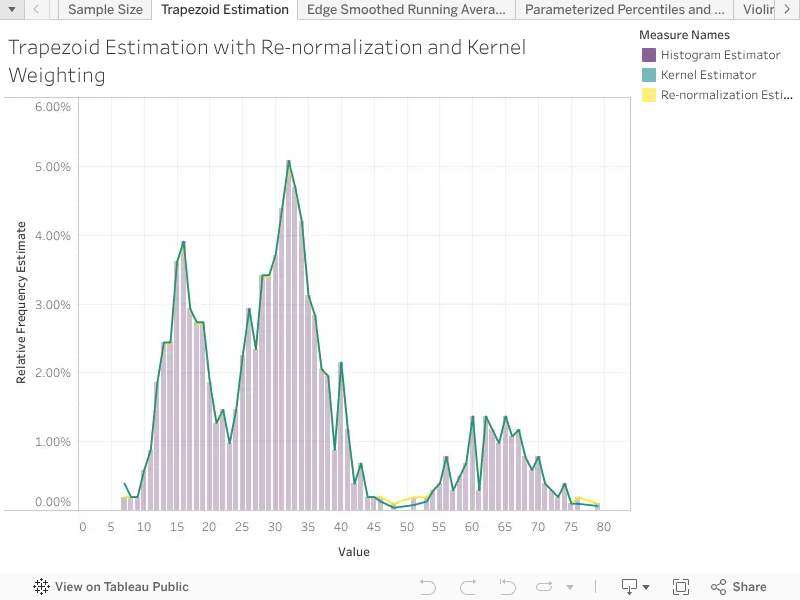# Trapezoid Density Estimation

Version 11

Description:

When individual bins of a relative frequency histogram are assumed to integrate to the relative frequency of the bin (density, or percentage of observations) then Tableau's bar style marks provide a mathematically consistent representation of the density estimator, in that the histogram will always integrate to one. However, Tableau's line style marks are often used to represent relative frequencies, which is equivalent to the trapezoid rule of integration. In this case of trapezoid estimation, the areas of the right trapezoids under the linear curves between consecutive points will not integrate to one. There are two methods to restore consistency, re-normalization and kernel weighting. Both techniques have appropriate use depending on the nature of the data and the information that needs to be represented. Re-normalization is appropriate when the representation needs to preserve the ratios between the relative heights of the points, and works well for data with a large amount of degeneracy, when point aggregates many records. In contrast when the data is relatively sparse so that most points aggregate over a single record, then the relative distance are an important representation of density, in this case kernel weighting is appropriate.

Re-normalizing divides each point on the curve by an appropriate constant of integration to ensure the curve integrates to 1.

Re-normalization can be accomplished through a table calculation using LOOKUP to determine the area of the individual right trapezoids between consecutive points, and TOTAL to find the total area. This calculation requires a continuous dimension [ORDER] that determines the position of the points, and any expression that can be used in a table calculation [AGGREGATE] that determines the height at each point.

Kernel weighting exploits basic Euclidean Geometry to recognize that the integration of the trapezoids is exactly equal to the integration of the rectangles that have the edges half way between each point. In this case each point is divided by half the distance between the previous point and the next point, except the edges which are divide by half the distance to the closest interior point

Kernel weighting is slightly easier to implement because it only requires the use of the LOOKUP table functions.

Example Calculation:

When re-normalizing we need to remember that TOTAL cannot accept a table calculation as an input so we will use WINDOW_SUM instead.

// Starting on the second point find the area of each right trapezoid,

// and then re-normalize by the total area.

[NORMALIZED] := 2 * [AGGREGATE] / WINDOW_SUM(

IIF(

INDEX() > 1,

(ATTR([ORDER]) - LOOKUP(ATTR([ORDER]), -1)) *

([AGGREGATE] + LOOKUP([AGGREGATE], -1)),

0

)

)

If the frequencies are derived from record counts we can take advantage of the cancellation of the total number of records terms from the numerator and denominator.

// Starting on the second point find the area of each right trapezoid,

// and then re-normalize by the total area.

[NORMALIZED] := 2 * SUM([Number of Records]) / WINDOW_SUM(

IIF(

INDEX() > 1,

(ATTR([ORDER]) - LOOKUP(ATTR([ORDER]), -1)) *

(SUM([Number of Records]) + LOOKUP(SUM([Number of Records]), -1)),

0

)

)

When kernel weighting we can use IFNULL to capture the edges of the table.

// Divide each point by the half width of the bar at that point

[NORMALIZED] := 2 * [AGGREGATE] / (

IFNULL(LOOKUP(ATTR([ORDER]), 1), ATTR([ORDER])) -

IFNULL(LOOKUP(ATTR([ORDER]), -1), ATTR([ORDER]))

)

Inputs and Setup:

Normalization requires any non-negative aggregate expression that can be passed to a table function, to determine the heights of the trapezoids, in this example called [AGGREGATE].

Re-normalization of the trapezoid density estimation requires a continuous ordered dimension along which the distribution is integrated, in this example the field is called [ORDER].

There reason that the first point is skipped when re-normalizing is that if there are N points along the dimension of integration then there will be N-1 trapezoids. Re-normalization can be re-written to use the first point and skip the last point, in which case the LOOKUP would have to look one point forward instead of backward.

Related Functions:

WINDOW_SUM, LOOKUP, ATTR, INDEX,IFNULL